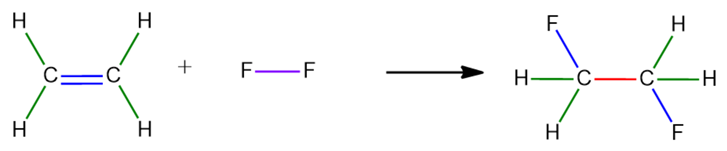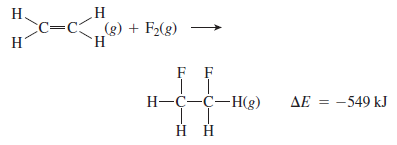# Problem: Consider the following reaction:Estimate the carbon–fluorine bond energy, given that the C—C bond energy is 347 kJ/mol, the C=C bond energy is 614 kJ/mol, and the F—F bond energy is 154 kJ/mol.

###### FREE Expert Solution

In calculating the enthalpy change of a reaction using bond energies, the formula is:

∆H°rxn = BE(reactants) - BE(products)

The reaction is:In the reactant side,

• 1 C=C single bonds will break

• 4 C-H single bonds will break

• 1 F-F single bond will also break

Bond breaking is an endothermic process. Therefore ∆H° will be positive.

80% (494 ratings)###### Problem Details

Consider the following reaction:Estimate the carbon–fluorine bond energy, given that the C—C bond energy is 347 kJ/mol, the C=C bond energy is 614 kJ/mol, and the F—F bond energy is 154 kJ/mol.